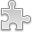# Courses / Module

Toggle Print

##LINEAR ALGEBRA 2

Module code: MT216C
Credits: 5
Semester: 2
Department: MATHEMATICS AND STATISTICS
International:Overview

Module Objective:
To introduce students to coordinate geometry in n-dimensions, vector spaces, linear transformations, eigenvalues and applications of linear algebra.

Definition of n x n determinant via permutations, determinants via expansion along a row or along a column, determinants via Gaussian elimination; inverses of n x n matrix via row reduction, mention adjoint formula and Cramer's rule.

Coordinate geometry and vectors in n-dimensions; norm, distance, dot product; triangle and parallelogram laws; angle, triangle and Cauchy-Schwartz inequalities; cross and triple product, volume of a paralleopiped.

Vector spaces with a focus on real vector spaces; linear span, linear independence, basis and dimension; linear maps and matrices; matrix multiplication as composition of linear maps; rank and nullity; rotations, reflections and orthogonal projections in 3 dimensions; Eigenvalues and eigenvectors of a matrix, diagonalization.

Applications, for example computer graphics, ranking in search engines, linear regression, Markov processes, Quantum systems. Computation using mathematical software.Learning OutcomesTeaching & Learning methodsAssessmentAutumn Supplementals/ResitsPre-RequisitesTimetable